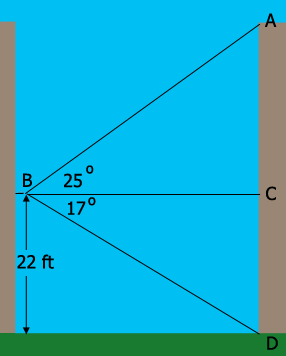SEARCH HOMEMath Central Quandaries & QueriesQuestion from Chelsey, a student: a person on a balcony of one building looks towards a second building. if the angle of elevation to the top of the second building is 25 degrees, the angle of depression to the bottom of the second building is 17 degrees, and the balcony of the first building is 22 feet above the ground, what is the height of the second building?Hi Chelsey,

You need to assume that the two buildings are built on level ground. Her is my diagram.The balcony is at point $B,$ 22 feet above the ground and $BC$ is a horizontal line segment. Since the buildings are built at the same level the distance from $D$ to $C$ is also 22 feet.

What trig function relates the measure of the angle $CBD$ (17 degrees) and the lengths of $CB$ and $DC?$ What is the distance from $C$ to $B?$

What trig function relates the measure of the angle $ABC$ (25 degrees) and the lengths of $CB$ and $AC?$ What is the distance from $A$ to $C?$

PennyMath Central is supported by the University of Regina and The Pacific Institute for the Mathematical Sciences.